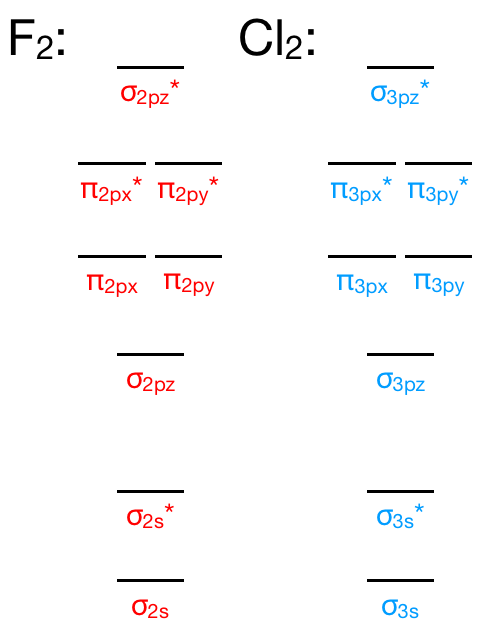# Problem: What is the bond order of Cl 2+ ?

###### FREE Expert Solution
90% (447 ratings)
###### FREE Expert Solution

We’re being asked to determine the bond order of Cl2+. For this, we need to do the following steps:

Step 1: Calculate the total number of valence electrons present.

Step 2: Draw the molecular orbital diagram.

Step 3: Calculate the bond order of the molecule/ion. Recall that the formula for bond order is:The molecular orbital diagram for Cl2 will be similar to that of F2:90% (447 ratings)###### Problem Details

What is the bond order of Cl 2+ ?

What scientific concept do you need to know in order to solve this problem?

Our tutors have indicated that to solve this problem you will need to apply the MO Theory: Bond Order concept. You can view video lessons to learn MO Theory: Bond Order. Or if you need more MO Theory: Bond Order practice, you can also practice MO Theory: Bond Order practice problems.

What is the difficulty of this problem?

Our tutors rated the difficulty ofWhat is the bond order of Cl 2+ ?...as low difficulty.

How long does this problem take to solve?

Our expert Chemistry tutor, Jules took 2 minutes and 52 seconds to solve this problem. You can follow their steps in the video explanation above.

What professor is this problem relevant for?

Based on our data, we think this problem is relevant for Professor Davis' class at UCF.